# Brain Teaser :: Can You Complete the SERIES?

in STEMGeeks3 months ago

Hey All; @StemGeeks Mathematician;

Without further, ado, let's get to our Brain Teaser :: Can You Complete the SERIES?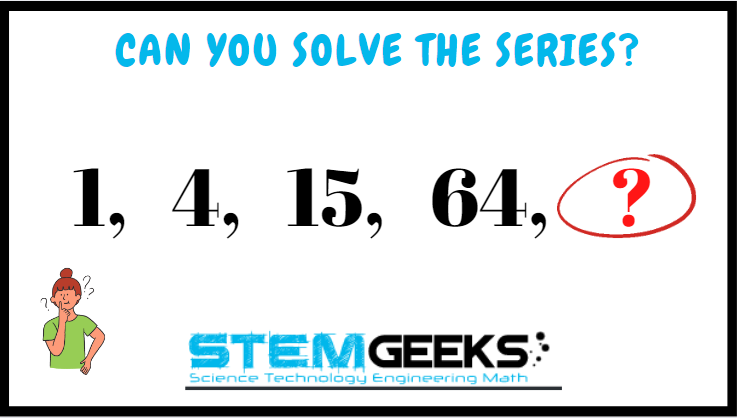Have a close look at the image given and see if you can solve the puzzle. This is not that difficult equation to solve and I assure that it should be FUN to solve this SERIES. Just remember there hasn't have to be a logic always.

There are few different solution and simple basic math's knowledge is required to get the equation right. Just give it a try - all you need to do is pick up a rough paper and see the equation that's being formed and you will get the answer. Try it out it should be FUN and its that simple I assure you. There is no right or wrong - comment your answer on the reply box below to enter a chance to WIN 10 STEM tokens...

### STEM token GiveAway...

I'll be again doing a giveaway of STEM tokens to the lucky random winner with the correct answer. For the last contest, which was Brain Teaser :: Can You FIND the Missing Number?.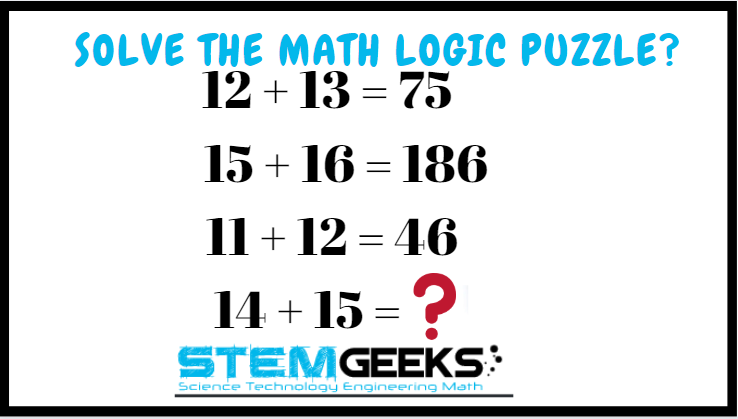### Solution :: Brain Teaser :: Can You FIND the Missing Number?

#### x + y * 2nd Number’s Unit Place Digit; lets take the example for each rows

• Row 1 => x + y * 2nd Number’s Unit Place Digit = 12 + 13 * 3 = 25 * 5 = 75

• Row 2 => x + y * 2nd Number’s Unit Place Digit = 15 + 16 * 6 = 31 * 6 = 186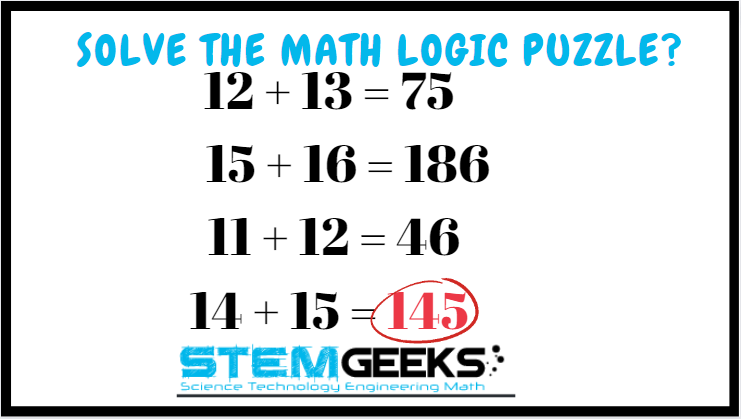• Row 3 => x + y * 2nd Number’s Unit Place Digit = 11 + 12 * 2 = 23 * 2 = 46

Now lets take the last row in question which is :: 14 + 15 = ?

• Row 4 => x + y * 2nd Number’s Unit Place Digit = 14 + 15 * 5 = 29 * 5 = 145

Finally the answer to the puzzle was:: 145

I guess this was a tuff problem to solve and we had just six entries for the same. And all of the entries provided the right the right answer.. I am going to use a random picker tool to pick a random winner.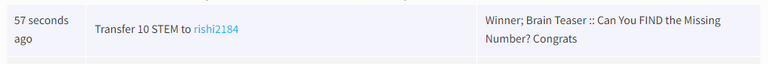Congratulations @rishi2184 - You WIN yourself 10 STEM tokens each. You should be having the rewards in your STEM Wallet Soon.

### Math Quote for the Day::

Here is the motivation to solve the Math Puzzle?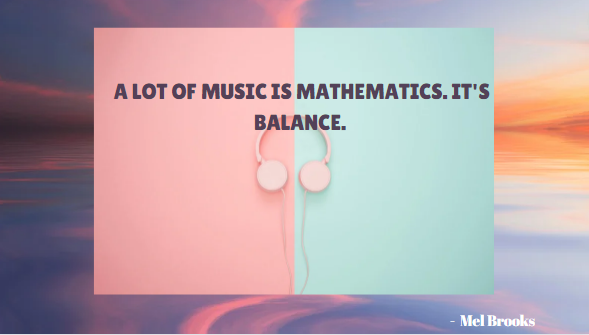If you like my work, then please spread the Word.. that we do have the Math Brain Teasers competition here @StemGeeks platform. Reblog is much appreciated.

Best RegardsFind Me on the Other Social Media Platforms::PS:: All the Maths Brain Teasers; are made by me using the Pro Canva License Version

Sort:

325

3 months ago

325
Pattern: 1, 4, 15, 64, 
1 = 1 x 1
2 = 2 (incrementing by 1) x (previous 1 x 1 +1) = 2 x 2 = 4
4 = 3 (incrementing by 1) x (previous 2 x 2 +1) = 3 x 5 = 15
16 = 4 (incrementing by 1) x (previous 3 x 5 +1) = 4 x 16 = 64
X = 5 (incrementing by 1) x (previous 4 x 16 +1) = 5 x 65 = 325

X = 325

Thank you very much! Glad to get chosen as the winner for the previous puzzle.

Congratulations, @gungunkrishu Your Post Got 95.3% Boost.
@gungunkrishu Burnt 25 UPME & We Followed That Lead.